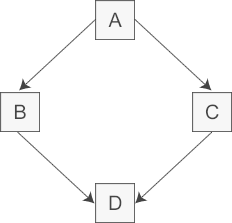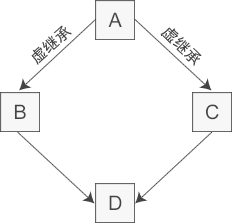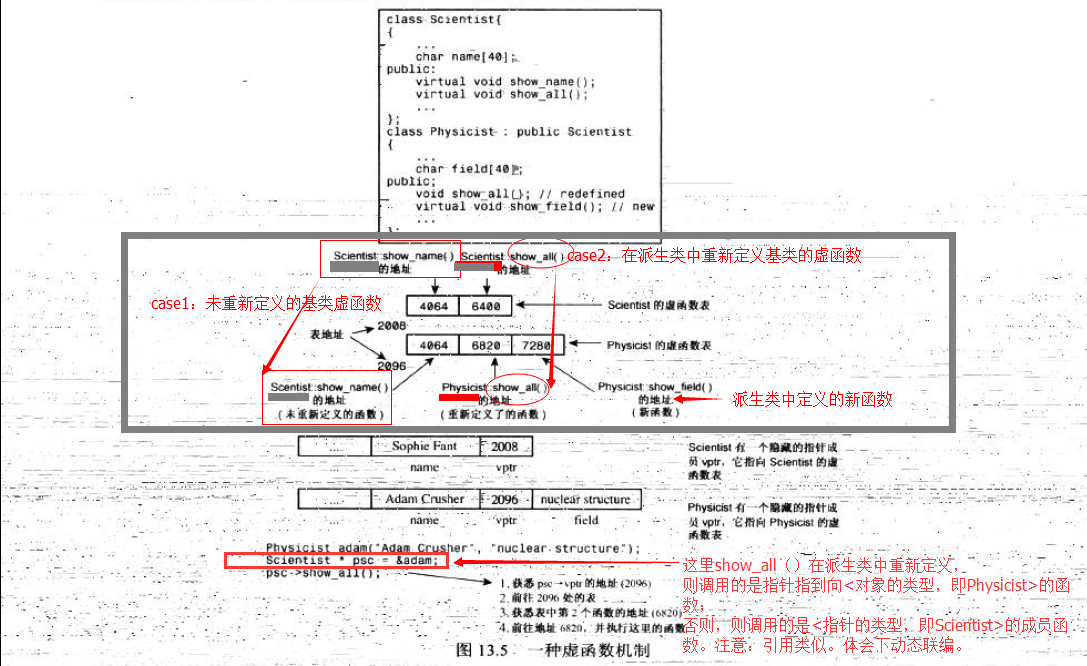﻿• 价格透明
• 信息保密
• 进度掌控
• 售后无忧# 1. 虚基类

## 1.1 虚基类作用

• 建立对象时所指定的类称为最远派生类。
• 虚基类的成员是由最远派生类的构造函数通过调用虚基类的构造函数进行初始化的。
• 在整个继承结构中，直接或间接继承虚基类的所有派生类，都必须在构造函数的成员初始化表中为虚基类的构造函数列出参数。如果未列出，则表示调用该虚基类的默认构造函数。
• 在建立对象时，只有最远派生类的构造函数调用虚基类的构造函数，其他类对虚基类构造函数的调用被忽略。

## 1.2 虚基类作用机制

### 1.2.1 不使用虚继承和虚派生的问题``````//间接基类A
class A{
protected:
int m_a;
};

//直接基类B
class B: public A{
protected:
int m_b;
};

//直接基类C
class C: public A{
protected:
int m_c;
};

//派生类D
class D: public B, public C{
public:
void seta(int a){ m_a = a; }  //命名冲突
void setb(int b){ m_b = b; }  //正确
void setc(int c){ m_c = c; }  //正确
void setd(int d){ m_d = d; }  //正确
private:
int m_d;
};

int main(){
D d;
return 0;
}``````

``void seta(int a){ B::m_a = a; }``

``void seta(int a){ C::m_a = a; }``

### 1.2.2虚继承和虚派生解决变量冗余

``````//间接基类A
class A{
protected:
int m_a;
};

//直接基类B
class B: virtual public A{  //虚继承
protected:
int m_b;
};

//直接基类C
class C: virtual public A{  //虚继承
protected:
int m_c;
};

//派生类D
class D: public B, public C{
public:
void seta(int a){ m_a = a; }  //正确
void setb(int b){ m_b = b; }  //正确
void setc(int c){ m_c = c; }  //正确
void setd(int d){ m_d = d; }  //正确
private:
int m_d;
};

int main(){
D d;
return 0;
}``````## 1.3 虚基类成员的可见性

• 如果 B 和 C 中都没有 x 的定义，那么 x 将被解析为 A 的成员，此时不存在二义性。
• 如果 B 或 C 其中的一个类定义了 x，也不会有二义性，派生类的 x 比虚基类的 x 优先级更高。
• 如果 B 和 C 中都定义了 x，那么直接访问 x 将产生二义性问题。

# 2. 虚函数

## 2.2 虚函数动态绑定演示

``````class A{
private:
int i;
public:
A();
A(int num) :i(num) {};
virtual void fun1();
virtual void fun2();

};

class B : public A{
private:
int j;
public:
B(int num) :j(num){};
virtual void fun2();// 重写了基类的方法
};

// 为方便解释思想，省略很多代码

A a(1);
B b(2);
A *a1_ptr = &a;
A *a2_ptr = &b;

// 当派生类“重写”了基类的虚方法，调用该方法时
// 程序根据 指针或引用 指向的  “对象的类型”来选择使用哪个方法
a1_ptr->fun2();// call A::fun2();
a2_ptr->fun2();// call B::fun1();
// 否则
// 程序根据“指针或引用的类型”来选择使用哪个方法
a1_ptr->fun1();// call A::fun1();
a2_ptr->fun1();// call A::fun1();``````

### 2.3 虚函数底层实现机制## 2.5 虚函数注意事项

（1） 基类方法中声明了方法为虚后，该方法在基类派生类中是虚的。
（2） 若使用指向对象的引用或指针调用虚方法，程序将根据对象类型来调用方法，而不是指针的类型。
（3）如果定义的类被用作基类，则应将那些要在派生类中重新定义的类方法声明为虚。

# 3. 纯虚函数

## 3.1 纯虚函数作用

1，当想在基类中抽象出一个方法，且该基类只做能被继承，而不能被实例化；

2，这个方法必须在派生类(derived class)中被实现；### 低价透明### 金牌服务### 信息保密